In :
import pymc as pm
import matplotlib.pyplot as plt
import matplotlib as mlp
import numpy as np
import pandas as pd

%matplotlib inline

In :
from IPython.display import Image

Out:As anonymous functions (like #+1 & in Mathematica), lambda functions in Python provide useful, but limited functional programming. They are used below to define the deterministic relations within a pymc graph.

In [ ]:


In :
# Initialization
observed_values = [1.]

rain = pm.Bernoulli('rain', .2, value=np.ones(len(observed_values)))

#Deterministic
p_sprinkler = pm.Lambda('p_sprinkler', lambda rain=rain: np.where(rain, .01, .4))

# "Real" sprinkler varible
sprinkler = pm.Bernoulli('sprinkler', p_sprinkler, value=np.ones(len(observed_values)))

p_grass_wet = \
pm.Lambda('p_grass_wet', lambda sprinkler=sprinkler, rain=rain: np.where(sprinkler, np.where(rain, .99, .9),
np.where(rain, .8, 0.0)))
grass_wet = pm.Bernoulli('grass_wet', p_grass_wet, value=observed_values, observed=True)

model = pm.Model([grass_wet, p_grass_wet, sprinkler, p_sprinkler, rain])


You can confirm that the lambda functions are current with:

In :
g = lambda sprinkler=sprinkler, rain=rain: np.where(sprinkler, np.where(rain, .99, .9), np.where(rain, .8, 0.0))
print(g(0,0),g(0,1),g(1,0),g(1,1))

(array(0.0), array(0.8), array(0.9), array(0.99))

In :
mcmc = pm.MCMC(model)
mcmc.sample(10000, 2000,2)

 [-----------------100%-----------------] 10000 of 10000 complete in 1.8 sec
In :
trace_r = mcmc.trace('rain')[:]
trace_p_sprinkler = mcmc.trace('p_sprinkler')[:]
trace_sprinkler = mcmc.trace('sprinkler')[:]
trace_p_grass_wet = mcmc.trace('p_grass_wet')[:]

In :
np.shape(trace_sprinkler)

Out:
(4000, 1)
In [ ]:


In :
trace_p_grass_wet = mcmc.trace('p_grass_wet')[:]

In :
pm.Matplot.plot(mcmc)

Plotting p_sprinkler_0
Plotting sprinkler_0
Plotting rain_0
Plotting p_grass_wet_0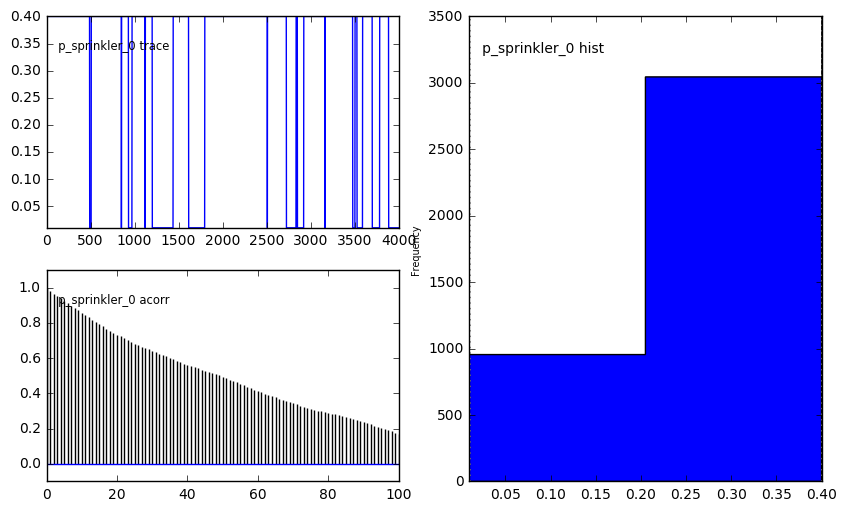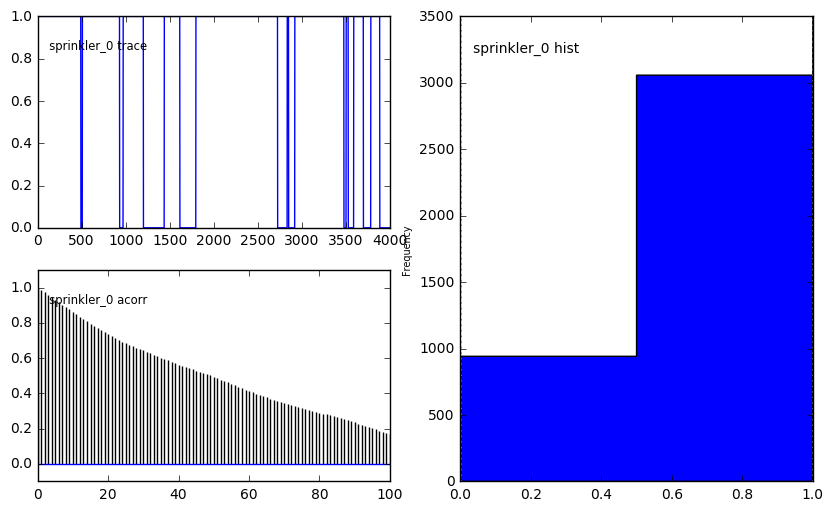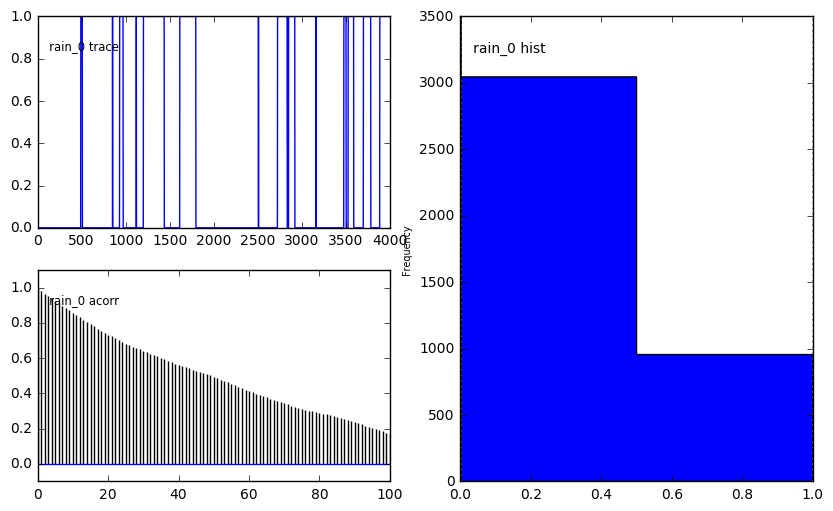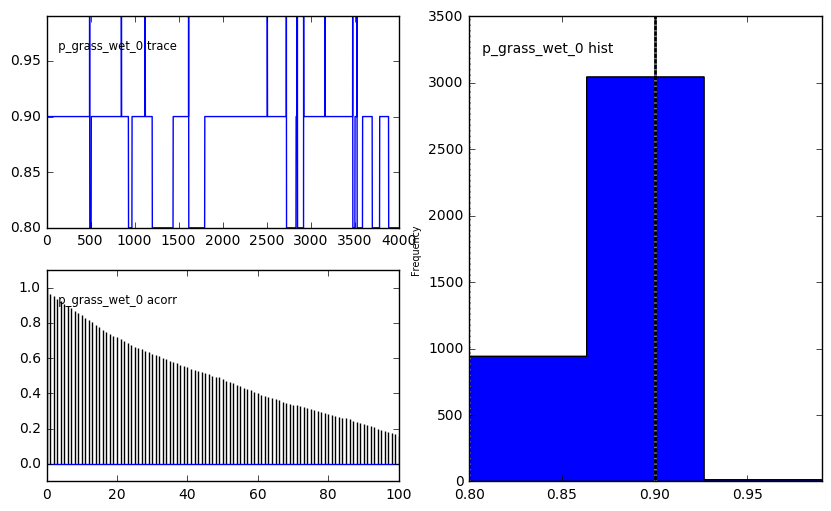Define a dictionary, and use pands to set up a data structure where you can now query the model.

In :
dictionary = {
'Rain': [1 if ii else 0 for ii in trace_r.tolist() ],
'Sprinkler': [1 if ii else 0 for ii in trace_sprinkler.tolist() ],
'Sprinkler Probability': [ii for ii in trace_p_sprinkler.tolist()],
'Grass Wet': [ii for ii in trace_p_grass_wet.tolist()],
}
df = pd.DataFrame(dictionary)

In :
#Given grass is wet, what is the probability that it  rained?

p_rain_wet = float(df[(df['Rain'] == 1) & (df['Grass Wet'] > 0.5)].shape) / df[df['Grass Wet'] > 0.5].shape
print(p_rain_wet)

0.38125

In :
#Given grass is wet, what is the probability that sprinkler was opened?
p_sprinkler_wet = float(df[df['Sprinkler'] == 1].shape) / df[df['Grass Wet'] > 0.5].shape
print(p_sprinkler_wet)

0.623

In :
#Is the grass wet if no rain and no sprinkler?

p_not_sprinkler_rain_wet = float(df[(df['Sprinkler'] == 0) & (df['Rain'] == 0)].shape) / df[df['Grass Wet'] > 0.5].shape
print(p_not_sprinkler_rain_wet)

0.0

In [ ]:


In :
from IPython.display import Image

#The ellipses are random variables. The triangles represent deterministic functions.

pm.graph.dag(pm.MCMC(model)).write_png('dag.png')
Image('dag.png')

Out: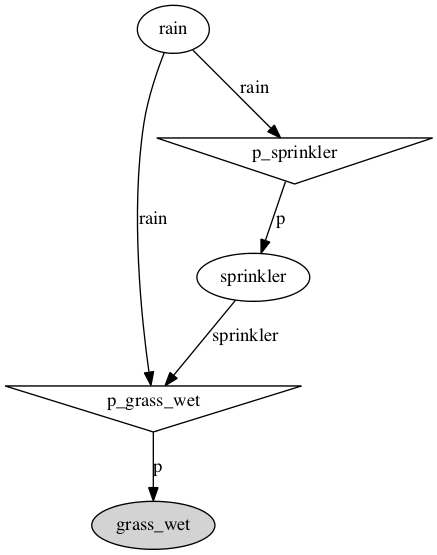In [ ]: